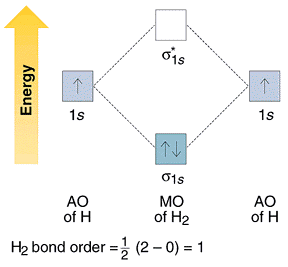# In the Lewis structure for barium fluoride, why does barium lose its valence electrons?

I searched for $$\ce{BaF2}$$ Lewis structure, and found out that the Barium atom loses its 2 valence electrons to form $$\ce{F- Ba^2+F-}$$.

Before seeing it I thought each of $$\ce{Ba}$$'s valence electrons form a single bond with each of the fluoride atoms $$\ce{F-B-F}$$ which also completes the octet for F atoms, and the formal charge in this case will be $$7-6-\frac{1}{2}(2) =0$$ for F atoms and $$2-0-\frac{1}{2}[2\cdot 2] = 0$$ for Ba, which works out.

I'm looking for an explanation on why Ba loses its electrons to form $$\ce{F- Ba^2+F-}$$ instead of $$\ce{F-Ba-F}$$.

• You've just stumbled upon an ionic compound. Prepare to see them every now and then, for they make up a sizeable portion of chemical universe. Come to think of it, how could you not notice NaCl, another example of those? Jun 6, 2019 at 8:54
• @IvanNeretin I am totally new to this subject, I'll search to understand what ionic compound is. Jun 6, 2019 at 9:40

First, a little bit of background. I assume you're already familiar with atomic orbitals — functions that describe the wave-like behavior of an electron in an atom. There's also molecular orbitals (MO), that describe its behavior in a molecule. You can ignore the odd-looking labels in images and such, since they're not entirely relevant to this question.

Atomic orbitals interact to form molecular orbitals (so called LCAO). The number of resulting molecular orbitals is the same as the number of the atomic orbitals that interact in the first place. To visualize this in simple interactions, you can use a molecular orbital diagram, in which orbitals of lower energy are drawn as lines placed lower than the others. There are three types of resulting MOs:

• Bonding MO, which is usually much lower in energy than the starting atomic orbitals
• Anti-bonding MO, which is usually much higher in energy
• Nonbonding MO, which would have the same energy as the starting atomic orbitals

You can imagine that electrons are rearranged when a molecule is formed, and they fill the orbitals with the lowest energies first, so the order is bonding first and anti-bonding last. In a symmetric molecule, like the boilerplate example $$\ce{H2}$$, this is what the diagram looks like:Image taken from this helpful page on Libretexts

# _________________________________________

Back to your question, the energy difference between the atomic orbitals and the bonding orbital (presented as the space between the lower bonding MO and the higher atomic orbitals of each H atom) is the same, so they're said to 'contribute the same to the bonding MO'. Bonds between homonuclear diatomic molecules are therefore considered to be purely covalent (whoops, almost!). Covalent compounds are the ones you're taught to draw Lewis structures for, apply formal charges, etc., but it's only one extreme of the spectrum.

The next example would be the MO diagram of HCl.Cl's 3p atomic orbitals are lower in energy (check the provided link for the reasoning); this time round, the atomic orbitals do not contribute equally to the molecular orbital, and Cl's orbital's contribution to the bonding orbital is greater. Electronegative atoms are generally said to have atomic orbitals of lower energy. This is probably more simply and familiarly stated as "the more electronegative atom pulls/attracts the bonding electrons". Note that, now, there's also an energy gap between the initial atomic orbitals. The $$\ce{H-Cl}$$ bond is, indeed, somewhere between covalent and the other extreme.

Now to the punchline, what happens when the gap is too large? This would be what an MO diagram of LiF would look like:Thanks Geoff!

The gap is so large that, essentially, the energy difference between molecular orbitals filled with electrons, and fluorine's atomic orbitals would be very small compared to the energy difference between F's 2p orbitals and Li's 2s. Put in simpler terms, the very electronegative fluorine has such a stronger pull than Li that the electrons are almost fully 'localized' on the fluorine, and Li has approximately lost its electron. This is what we call an ionic bond, the other end of the spectrum.

Most bonds between very electronegative atoms and very electropositive ones are considered ionic, and there's the guideline that metal-nonmetal bonds are often ionic. Don't let it trip you up though, since it's a vague general rule, with plenty of exceptions. As you might have suspected, there's no truly, purely ionic bond either, and it's a spectrum.